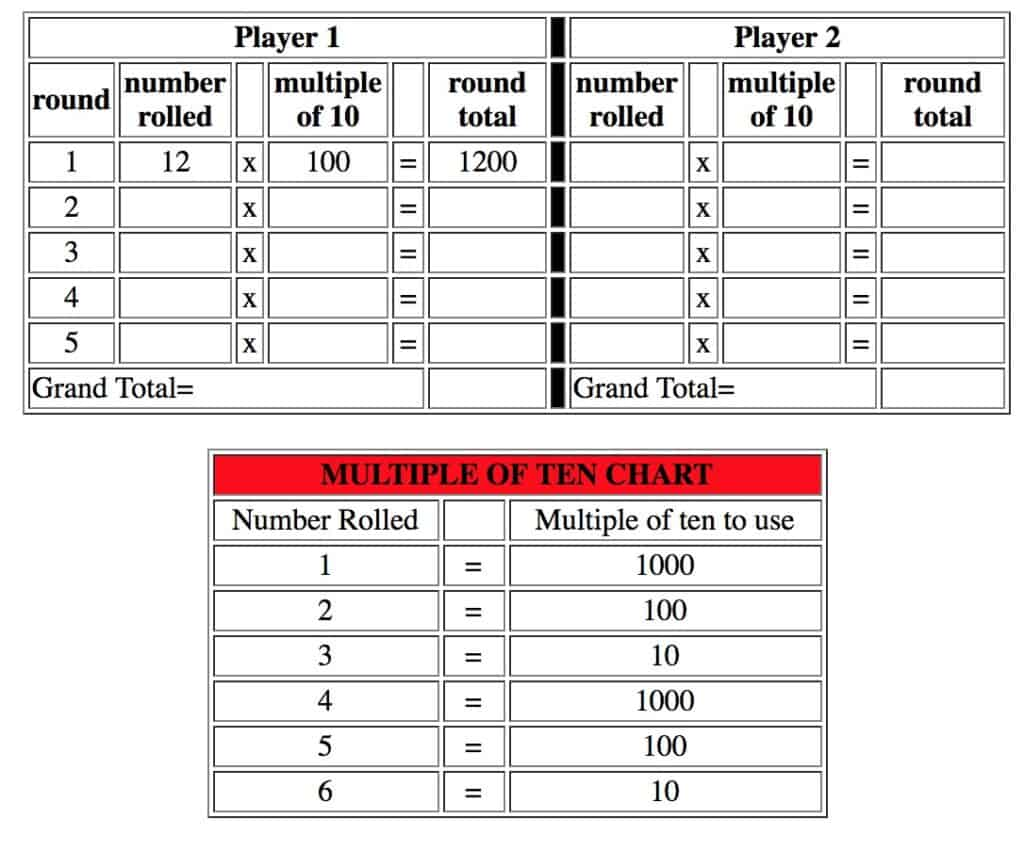# Mr. R.’s World of Math

## multiples of ten game

Lesson: Multiples of Ten Game
Skills: facility with multiplying numbers by ten and other powers of ten

Notes: Before playing this game, students should be aware that when a number is multiplied by ten, a zero is added to the end of the number:

### 5 X 10 = 50

Or by one hundred, two zeroes are added:

### 5 X 100= 500

Procedure:

1. First player rolls two dice and writes the product in the ‘number rolled’ column.

2. First player then rolls one die to get multiple of ten. For example, if they roll a 1, they look at multiple of tens chart (see below) and find that 1 will give them 1000. They write 1000 in the multiple of ten column.

3. They find product and write it in for round total.

Example: first player has rolled a 3 and 4 on first roll, so they multiply and write a 12 in ‘number rolled’ column, then they roll a 2, so chart shows that a 2 gets them 100. The 100 is written into the ‘multiple of ten’ column. The round total is then 1200.4. Second player rolls the dice and completes their first round.

5. After all rounds are complete, grand totals are figured by adding scores from each round and player with higher sum wins.